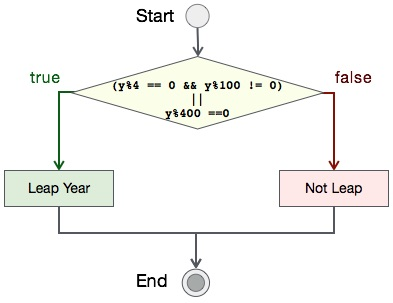# Leap Year Program In C

Finding a year is leap or not is a bit tricky. We generally assume that if a year number is evenly divisible by 4 is leap year. But it is not the only case. A year is a leap year if −

• It is evenly divisible by 100

• If it is divisible by 100, then it should also be divisible by 400

• Except this, all other years evenly divisible by 4 are leap years.

Let's see how to we can create a program to find if a year is leap or not.

## Algorithm

Algorithm of this program is −

```START
Step 1 → Take integer variable `year`
Step 2 → Assign value to the variable
Step 3 → Check if `year` is divisible by 4 but not 100, DISPLAY "leap year"
Step 4 → Check if `year` is divisible by 400, DISPLAY "leap year"
Step 5 → Otherwise, DISPLAY "not leap year"
STOP
```

## Flow Diagram

We can draw a flow diagram for this program as given below −## Pseudocode

The pseudocode of this algorithm sould be like this −

```procedure leap_year()

IF year%4 = 0 AND year%100 != 0 OR year%400 = 0
PRINT year is leap
ELSE
PRINT year is not leap
END IF

end procedure
```

## Implementation

Implementation of this algorithm is given below −

```#include <stdio.h>

int main() {
int year;
year = 2016;

if (((year % 4 == 0) && (year % 100!= 0)) || (year%400 == 0))
printf("%d is a leap year", year);
else
printf("%d is not a leap year", year);

return 0;
}
```

## Output

Output of the program should be −

```2016 is a leap year
```
simple_programs_in_c.htm